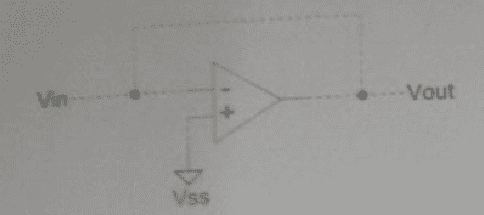# Input and Output Impedance of an Opamp Circuit

• Engineering
Homework Statement:
What is input and output impedance of opamp in circuit
Relevant Equations:
Input impedance is infinity, output impedance is 0I know for ideal opamp the input Z is infinite, and output Z is 0, but not sure about this opamp.

berkeman
Mentor
Problem Statement: What is input and output impedance of opamp in circuit
Relevant Equations: Input impedance is infinity, output impedance is 0

View attachment 244354
I know for ideal opamp the input Z is infinite, and output Z is 0, but not sure about this opamp.
The picture is very hard to see. Is is connected as a simple follower?

And are you asked to assume an ideal opamp, or are you using a datasheet for a real part to find those impedances?

•jaus tail
Yes there is a voltage follower. There isn't any data sheet. Just the figure n we have to write the input and output impedance.

jaus tai - you clearly have to discriminate between (a) an opamp and (b) an amplifier circuit that is build using an opamp as a core.
Your circuit is not a pure (naked) opamp but a "circuit" (an amplifier) because we have negative feedback, which DRASTICALLY changes the input as well as output resistances.

Just a basic question (which may help you to find the answer for the input resistance): As long as the opamp is operated within its linear transfer characteristic and with a finite output voltage smaller than the supply voltage, what do you think could be the approximate diff. voltage between the inv. and the non-inv. input terminals (µV, mV, V )?

Approximately the voltage difference would be 0 between the inverting and non-inverting terminal
How do I find the input and output impedance since there's no resistance vin circuit. No feedback resistance too. Even that's a simple short circuit.

From this link I guess the input impedance would be very high. It'd be like Rin times the Open loop gain. and Output impedance is low. I don't understand how the output impedance would be low.
https://www.electronics-tutorials.ws/opamp/opamp_3.htmlRef:
Electronics-Tutorials-Opamp

When the voltage difference between both inputs is nearly zero (for the model of an IDEAL opamp it is identical to zero), what then is the resistance between the input signal source and ground?

When the voltage difference between both inputs is nearly zero (for the model of an IDEAL opamp it is identical to zero), what then is the resistance between the input signal source and ground?
The resistance between input signal source and ground would be same as resistance between signal source and input of opamp, which is zero here. Now since the non-inverting terminal is grounded, then the inverting terminal is also grounded, so the entire input signal shorts to the ground?? But the input impedance of opamp is very high.

I went through this link that says the input impedance is R1.
https://www.electronics-notes.com/a...rational-amplifier-op-amp/input-impedance.php
Ref: Opamp Input Impedance
There is an inverting opamp circuit with imput Z = R1.

Tom.G
But the input impedance of opamp is very high.
Yes, the input impedance of the op-amp itself is high... and it is connected in parallel with the output impedance which is low (almost Zero).

So what is the the parallel impedance of a high value resistor paralleled by a Zero Ohm resistor?

Yes, the input impedance of the op-amp itself is high... and it is connected in parallel with the output impedance which is low (almost Zero).

So what is the the parallel impedance of a high value resistor paralleled by a Zero Ohm resistor?
Zero ohm resistor. So the input impedance of circuit is 0 ohm and the output impedance is also 0 ohm.

•Tom.G
I went through this link that says the input impedance is R1.
https://www.electronics-notes.com/a...rational-amplifier-op-amp/input-impedance.php
Ref: Opamp Input Impedance
There is an inverting opamp circuit with imput Z = R1.

But in your circuit there is no resistor R1. If there would be one, why didnt you show it?
In this case, the answer would be different, of course.
Rule: A good and correct answer requires a good, clear and correct question.

But in your circuit there is no resistor R1. If there would be one, why didnt you show it?
In this case, the answer would be different, of course.
Rule: A good and correct answer requires a good, clear and correct question.
R1 is not in the question. I couldn't find the circuit in question, anywhere in the book about opamp. So I searched for input resistance of opamp and the link came up.
We can like assume R1 to be wire resistance and then consider it is equal to negligible i.e 0.
The original question is the one that I have pasted on the first post. No R1.

Sorry - I have responded to your post #8 in which you have mentioned R1.
I will not repeat such an error....sorry.
I withdraw....

Sorry - I have responded to your post #8 in which you have mentioned R1.
I will not repeat such an error....sorry.
I withdraw....
It's all right. No problem...